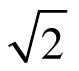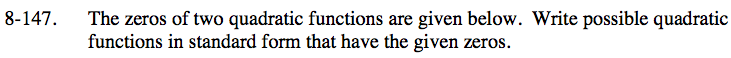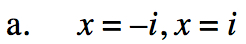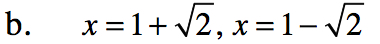### Home > INT3 > Chapter 8 > Lesson 8.3.4 > Problem8-147

8-147.
1. The zeros of two quadratic functions are given below. Write possible quadratic functions in standard form that have the given zeros. Homework Help ✎

1. x = –i, x = i

2. x = 1 +, x = 1 –$y=(x + i)(x - i)$$y = (x - (1 + \sqrt{2}))(x - (1 - \sqrt{2}))$